# JEE Main 2020 Chemistry Paper With Solutions Jan 9 Shift 1

JEE Main 2020 solved shift 1 Chemistry paper (9th January) is given here. Students can easily download these solutions in PDF format for free. Learning these solutions help students to get a knowledge of the type of questions asked for the JEE Main exam. Solutions are given in a step by step manner so that students can easily understand the problems.

### January 9 Shift 1 - Chemistry

Question 1. The de Broglie wavelength of an electron in the 4th Bohr orbit is:

1. a) 4πa0
2. b) 42πa0
3. c) 8πa0
4. d) 6πa0

Solution:

1. n=4

Z = 1 , λ= ?Circumference (2πr)= nλ

2πa0n2/z = n

On solving, we get

= 8πao

Question 2. If the magnetic moment of a dioxygen species is 1.73 B.M, it may be:

1. a) O2, O2-, or O2+
2. b) O2-, or O2+
3. c) O2 or O2-,
4. d) O2, O2+

Solution:

1.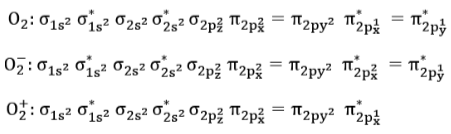Question 3. If enthalpy of atomisation for Br2(l) is x kJ/mol and bond enthalpy for Br2 is y kJ/mol, the relation between them:

1. a) is x > y
2. b) is x < y
3. c) is x = y
4. d) does not exist

Solution:

1.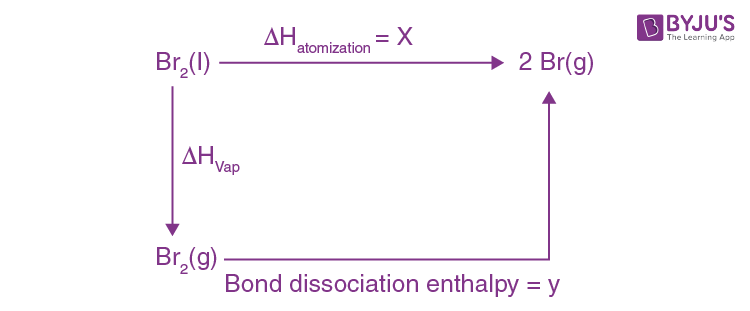ΔHatomisation = ΔHvap + y

x − y = ΔHvap

Question 4. Which of the following oxides are acidic, basic and amphoteric, respectively?

1. a) MgO, Cl2O, Al2O3
2. b) N2O3, Li2O, Al2O3
3. c) SO3, Al2O3, Na2O
4. d) P4O10, Cl2O, CaO

Solution:

1. Non-metallic oxides are acidic in nature, metallic oxides are basic in nature and Al2O3 is amphoteric in nature.

Question 5. Complex X of composition Cr(H2O)6Cln, has a spin only magnetic moment of 3.83 BM. It reacts with AgNO3 and shows geometrical isomerism. The IUPAC nomenclature of X is :

1. a) Hexaaqua chromium(III) chloride
2. b) Tetraaquadichlorido chromium(III) chloride dihydrate
3. c) Hexaaquachromium(IV) chloride
4. d) Tetraaquadichlorido chromium(IV) chloride dihydrate

Solution:

1. p>Spin only magnetic moment = 3.8 B. M.

This implies, µ = √(n(n + 2)) B.M. (√16 = 4 implies that √15 should be less than four.)

This means, n = 3 as √15 = √(3(3 + 2))

Cr (24) = [Ar]4s1 3d5 (g.s)

For 3 unpaired electrons, the oxidation state of Cr should be +3

Cr3+ can be attained if the complex has a structure that looks like: [Cr(H2O)4Cl2]Cl. 2H2O [Cr(H2O)4Cl2]Cl. 2H2O has the IUPAC name : Tetraaquadichloridochromium(III) chloride dehydrate.

Question 6. The electronic configuration of bivalent europium and trivalent cerium, are: (Atomic Number : Xe = 54, Ce = 58, Eu = 63)

1. a) [Xe]4f 7, [Xe]4f 1
2. b) [Xe]4f 76s2 , [Xe]4f 26s2
3. c) [Xe]4f 2, [Xe]4f 7
4. d) [Xe]4f 4, [Xe]4f 9

Solution:

1. Ce (58): [Xe] 6s24f 2 (g.s)

Ce3+: [Xe]4f1

Eu(63) ∶ [Xe]6s24f 7 (g.s)

Eu2+ ∶ [Xe]4f 7

Question 7. The Ksp for the following dissociation is = 1.6 × 10–5. PbCl2 (s) ⇌ Pb2 + (aq) + 2Cl-(aq). Which of the following choices is correct for a mixture of 300 mL 0.134 M Pb(NO3)2 and 100mL

1. a) Q > Ksp
2. b) Q < Ksp
3. c) Q = Ksp
4. d) Not enough data provided

Solution:

1. Pb(NO3)2: mmoles= 300 mL × 0.134 M = 40.2

NaCl: mmoles = 100 mL × 0.4 M = 40

This implies, [Pb]2+ = 40.2/400 ≈ 0.1M

Qsp = [Pb2+][2Cl]2 = 4 × 10−3 > Ksp

Question 8. The compound that cannot act both as oxidising and reducing agent is :

1. a) H2SO3
2. b) HNO2
3. c) H3PO4
4. d) H2O2

Solution:

1. When the oxidation state is maximum it acts like a strong oxidising agent.

When the oxidation state is minimum it acts like a strong reducing agent.

When the oxidation state is between its maximum and minimum, it acts like both an oxidizing and as a reducing agent.

In H3PO4, P has a +5 oxidation state and hence can act like a strong oxidising agent. In the rest, the oxidation state is between their maximum and minimum.

Question 9. B has a smaller first ionization enthalpy than Be. Consider the following statements:

(i) It is easier to remove 2p electron than 2s electron

(ii) 2p electron of B is more shielded from the nucleus by the inner core of electrons than the 2s electron of Be

(iii) 2s electron has more penetration power than 2p electron

Atomic radius of B is more than Be (Atomic number B=5, Be=4)

The correct statements are:

1. a) (i), (ii), and (iii)
2. b) (i), (iii), and (iv)
3. c) (ii), (ii), and (iii)
4. d) (i), (ii), and (iv)

Solution:

1. Be (4): 1s22s2

B (5): 1s22s22p1

The electron in 2p1 can easily be extracted.

The penetrating power is of the order: s > p > d > f The shielding power order: s > p > d > f

As we move along the period, the size decreases, as Zeff increases. Hence the radius of B is smaller than the radius of Be.

Question 10. [Pd(F)(Cl)(Br)(I)]2−,has n number of geometrical Isomers. Then, the spin-only magnetic moment and crystal field stabilisation energy [CFSE] of [Fe(CN)6]n−6 , respectively, [Note: Ignore pairing energy].

1. a) 1.73 BM and – 2Δ0
2. b) 2.84 BM and – 1.6Δ0
3. c) 0 BM and – 2.4Δ0
4. d) 5.92 BM and 0

Solution:

1.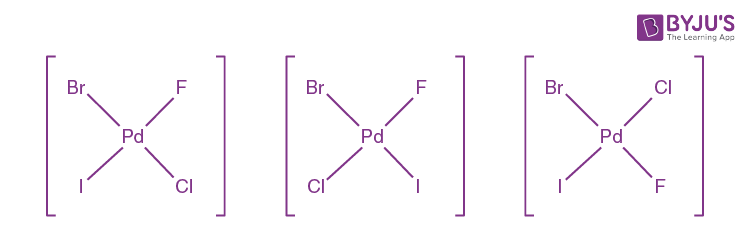Number of geometrical isomers (n) = 3

[Fe(CN)6]n−6 = [Fe(CN)6]3−6 = [Fe(CN)6]−3

This implies, that Iron is in its +3 oxidation state.

Fe3+(26): [Ar]3d5

CN is a strong ligand in [Fe(CN)6]−3 and causes pairing. Hence, according to CFT, the configuration will be t2g5 e0g.

Hence, there is only 1 unpaired electron, i.e, n=1 in √n(n + 2) = √3 =

1.73 B.M

CFSE = (−0.4 × nt2g + 0.6 × neg0

= (−0.4 × 5 + 0.6 × 0)Δ0

= -2Δ0

Question 11. According to the following diagram, A reduces BO2 when the temperature is: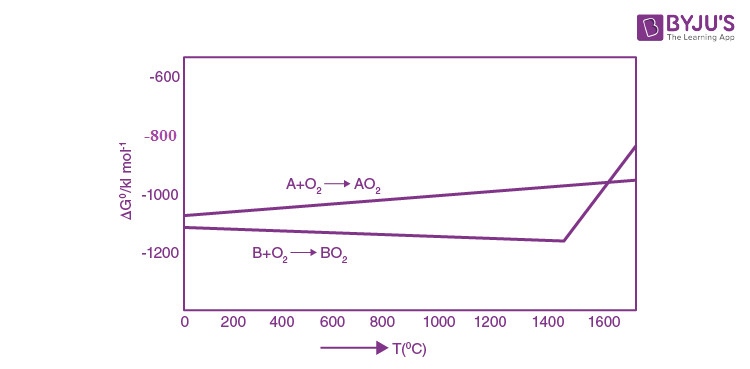1. a) > 14000C
2. b) < 14000C
3. c) > 12000C
4. d) < 12000C

Solution:

1. Solution: In Ellingham’s diagram, the line of the element that lies below can reduce the oxide of the element which lies above it. Therefore, for A to reduce BO2 , the temperature when the line for element A is below that of BO2, according to the graph when T > 1400 ℃.

For T > 1400 ℃ , ΔGr < 0 for A + BO2-> B + AO2

Question 12. For following reactions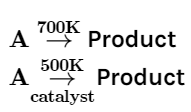It was found that the 𝐸𝑎 is decreased by 30 kJ/mol in the presence of catalyst. If the rate remains unchanged, the activation energy for catalysed reaction is (Assume pre exponenetial factor is same)

1. a) 75 kJ/mol
2. b) 135 kJ/mol
3. c) 105 kJ/mol
4. d) 198 kJ/mol

Solution:

1. K = Ae(-Ea/RT)

Kcatalyst = Kwithoutcatalyst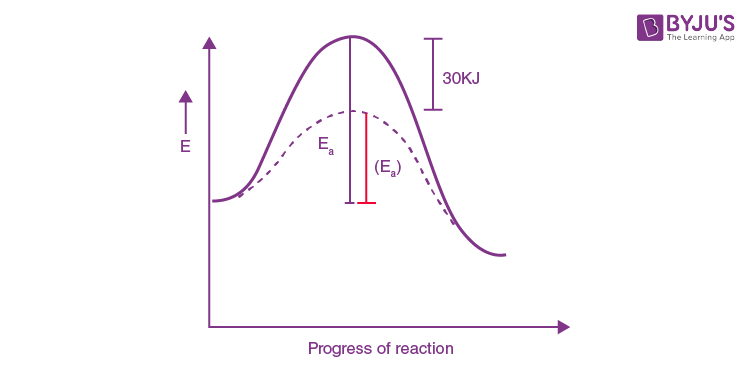$$Ae^{(-\frac{(Ea)c}{RT_{500k}})} = Ae^{(-\frac{(Ea)}{RT_{700k}})}$$

$$e^{(-\frac{(Ea)c}{RT_{500k}})} = e^{(-\frac{(Ea)}{RT_{700k}})}$$

$${-\frac{(Ea)c}{RT_{500k}}} = {-\frac{(Ea)}{RT_{700k}})}$$

Eac = Ea - 30

-(Ea-30)/T500k = -Ea/T700k

On solving Ea = 105 kJ mol-1

Question 13. ‘X’ melts at low temperature and is a bad conductor of electricity in both liquid and solid state. X is:

1. a) Mercury
2. b) Silicon Carbide
3. c) Zinc Sulphide
4. d) Carbon Tetrachloride

Solution:

1. CCl4 is non polar and does not conduct in either solid or liquid state.

Question 14. The major product Z obtained in the following reaction scheme is: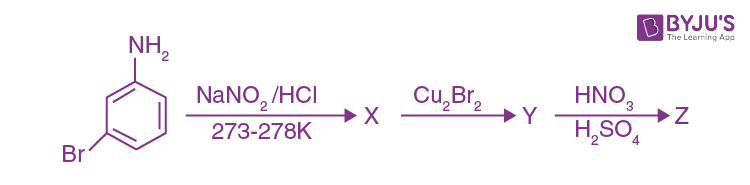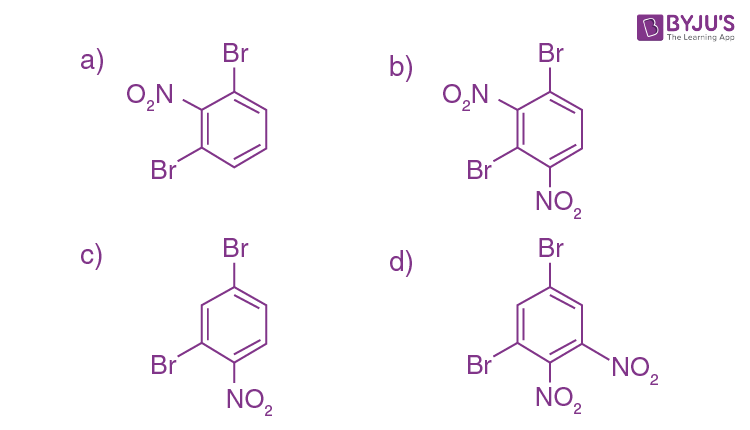Solution:

1.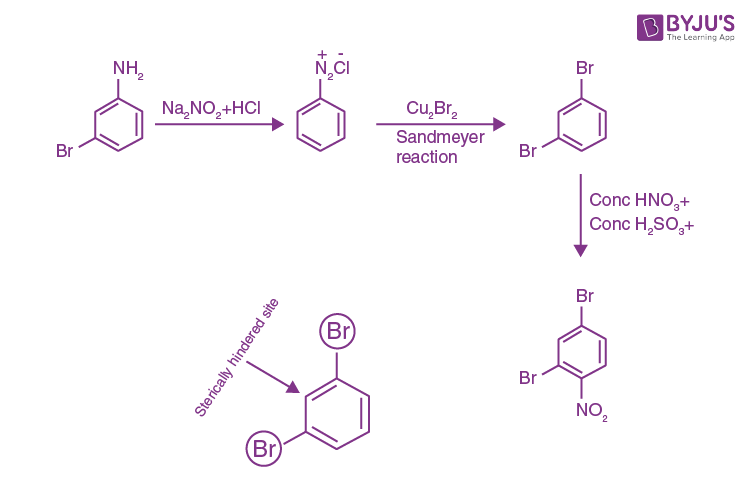Hence, major product formed is that of option c.

Question 15. Which of these will produce the highest yield in Friedel-Craft’s reaction?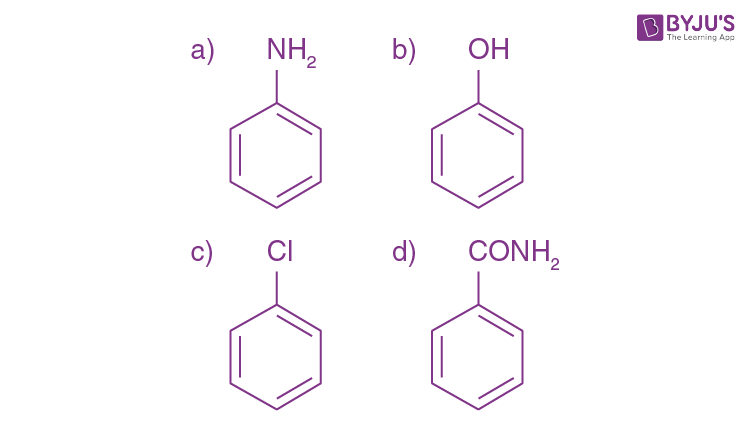Solution:

1. Out of the four options given, only aniline and phenol show strong +R effects, but as we know, aniline is a Lewis base and can react with a Lewis acid that is added during the reaction. Hence, Phenol gives the highest yield in Friedel-Craft’s reaction.

Question 16. The major product (Y) in the following reactions is :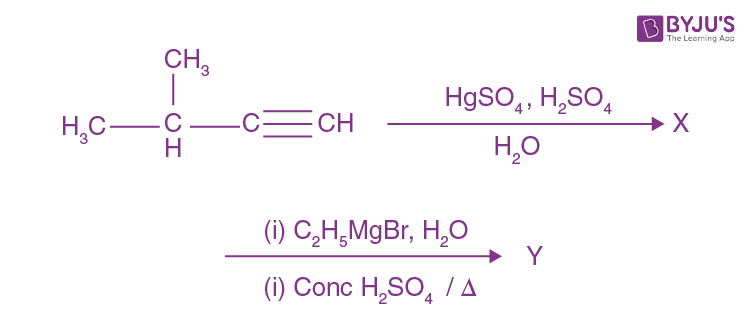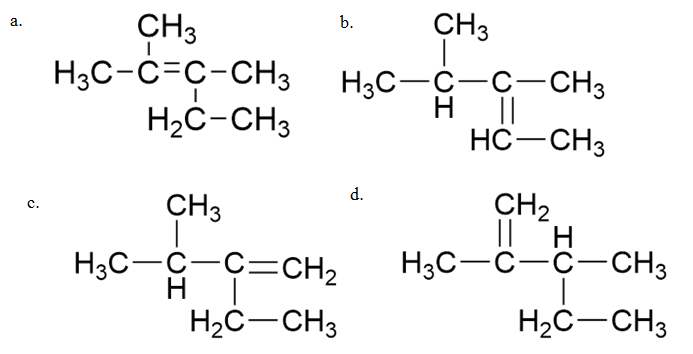Solution:

1.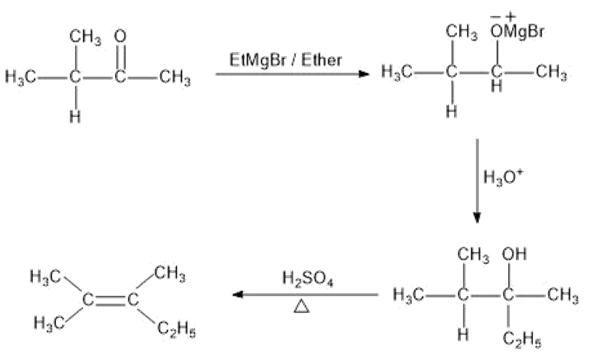Question 17. The correct order of heat of combustion for following alkadienes is: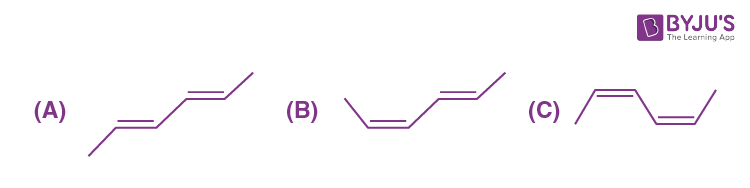1. a) C > B > A
2. b) B > A > C
3. c) A > B > C
4. d) C > A > B

Solution:

1. Heat of combustion ∝ 1/ stability

The trans-isomer is more stable than the cis-isomer. More the number of trans forms in a structure, higher the stability.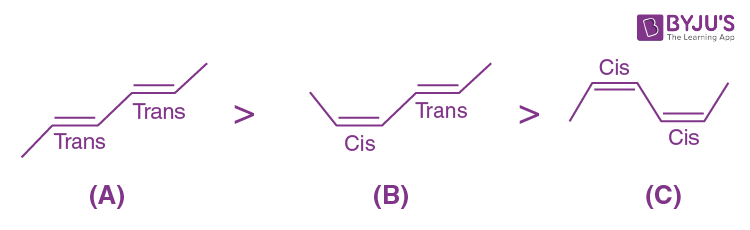Question 18. The increasing order of basicity for the following intermediates is (from weak to strong)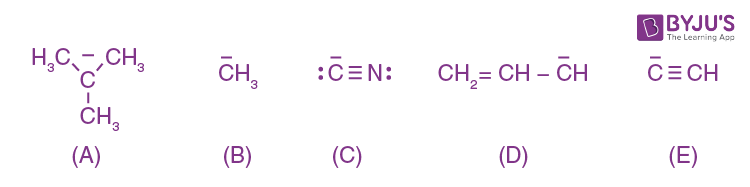1. a) A > B > D > E > C
2. b) B > A > D > C > E
3. c) A > B > E > D > C
4. d) C > E > D > B > A

Solution:

1. As we know weaker the conjugate base, stronger the acid.

The order of stability of conjugate base: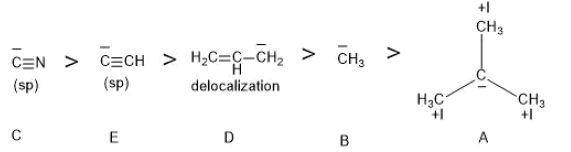Hence, the order of basicity or acidic strength is:

A > B > D> E> C

Question 19. A chemist has 4 samples of artificial sweetener A, B, C and D. To identify these samples, he performed certain experiments and noted the following observations:

(i) A and D both form blue-violet colour with ninhydrin.

(ii) Lassaigne extract of C gives positive AgNO3 test and negative Fe4[Fe(CN)6]3 test.

(iii) Lassaigne extract of B and D gives positive sodium nitroprusside test.

Based on these observations which option is correct?

1. a) A – Alitame, B – Saccharin, C – Aspartame, D – Sucralose
2. b) A –Saccharin, B – Alimate, C – Sucralose, D – Aspartame
3. c) A – Aspartame, B – Alitame, C – Saccharin , D – Sucralose
4. d) A – Aspartame, B – Saccharin, C – Sucralose, D – Alitame

Solution:

1.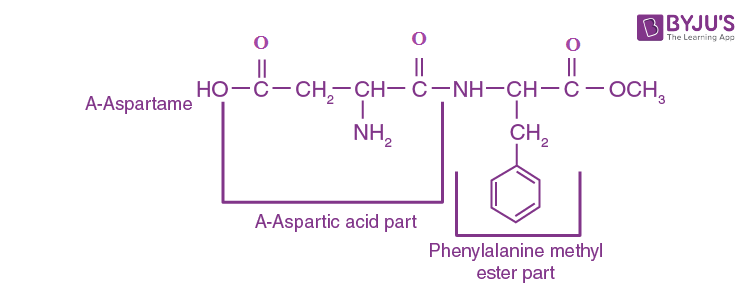It has a free amine group and hence reacts with ninhydrin to give a purple colour known as

Ruhemann's purple.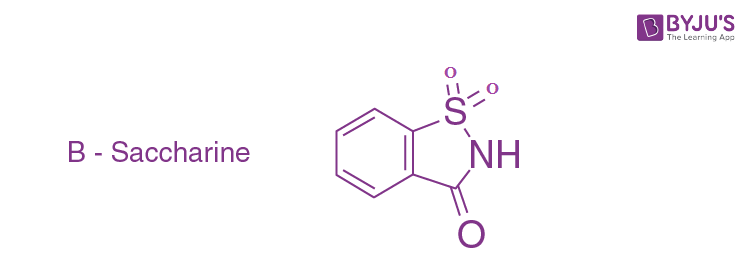It has Sulphur, therefore, it will give a positive test with sodium nitroprusside.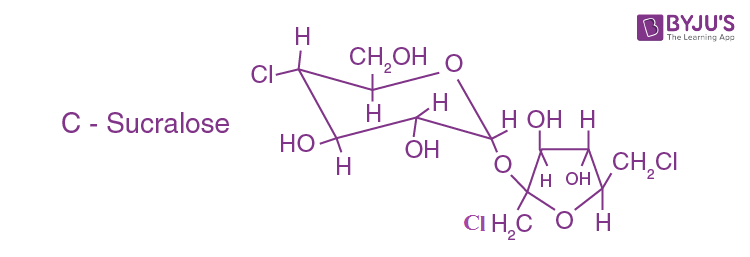It has chlorine and hence it forms a precipitate with AgNO3 in the Lassaigne’s extract of the sugar.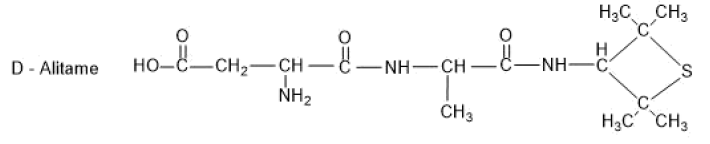It has a free amine group and hence reacts with ninhydrin to give purple colour known as Ruhemann's purple. Also, it has Sulphur, therefore, it will give positive test with sodium nitroprusside.

Question 20. Identify (A) in the following reaction sequence: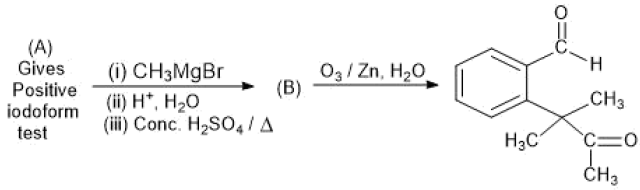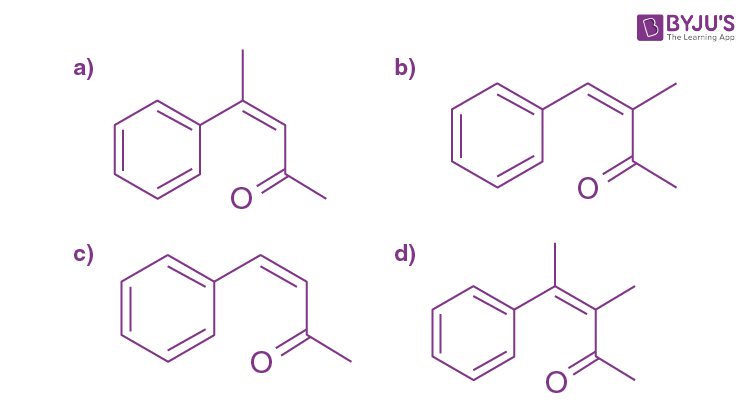Solution:

1.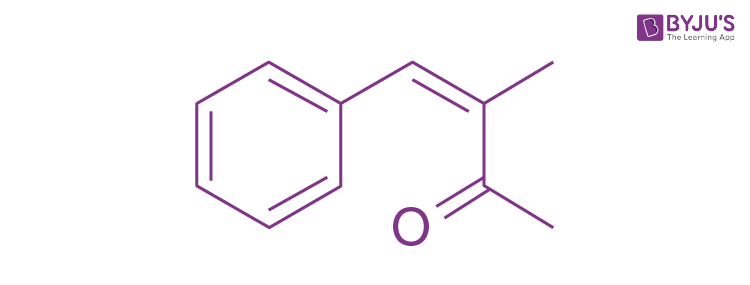is a methyl ketone, which gives positive Iodoform test.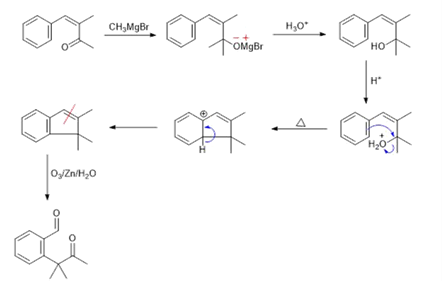Question 21. The molarity of HNO3 in a sample which has density 1.4 g/mL and mass percentage of 63% is :(Molecular weight of HNO3= 63).

Solution:

1. %w/w = 63%

ρ = 1.41g/mL

M = ((%w/w)× ρ× 10)/MM

= (63×1.4×10)/63

= 14 mol/L

Question 22.The hardness of a water sample containing 10-3 M MgSO4 expressed as CaCO3 equivalents (in ppm)is (molar mass of MgSO4 is 120.37 g/mol)

Solution:

1. Hardness of water is measured in ppm in terms CaCO3.

nCaCO3 = nMgSO4

ppm is the parts (in grams) present per million i.e, 106

1000 mL has 10−3 moles of MgSO4.

Grams of CaCO3 in 1000 mL = 10−3 ×100 grams

Grams of CaCO3 in 1 mL = 10−3×100/1000 grams

Hardness = (10−3×100/1000)×106

= 100

Question 23. How much amount of NaCl should be added to 600 g of water (ρ = 1.00 g/mL) to decrease the freezing point of water to -0.2°C? (The freezing point depression constant for water = 2 K Kg mol-1)

Solution:

1. NaCl is strong electrolyte and gives 2 ions in the solution. This implies, i = 2.

Molarity = (w×1000)/58.5×600

ΔTf = 0.20C

ΔTf = i ×kf ×m

On solving we get

w = 1.76 grams

Question 24. 108 g silver (molar mass 108 g mol-1) is deposited at cathode from AgNO3(aq) solution by a certain quantity of electricity. The volume (in L) of oxygen gas produced at 273K and 1 bar pressure from water by the same quantity of electricity is

Solution:

1. On applying Faraday’s 1st law,

Moles of Ag deposited= 108/108= 1 mol.

Ag+ + e- -> Ag

1Faraday is required to deposit 1 mole of Ag.

H2O -> 2H+ + ½ O2 + 2e-

½ moles of O2 are deposited by 2F of charge.

This implies, 1F will deposit ¼ moles of O2.

Using PV = nRT

P= 1 bar

T= 273 K

R= 0.0823 Lbar mol−1 K−1

On solving we get,

V = 5.68 L

Question 25. The mass percentage of nitrogen in histamine is:

Solution:

1. Molecular mass of Histamine= 111

In Histamine, 3 nitrogen atoms are present (42g)

The percentage of nitrogen by mass in Histamine = (42/111)×100 = 37.84%.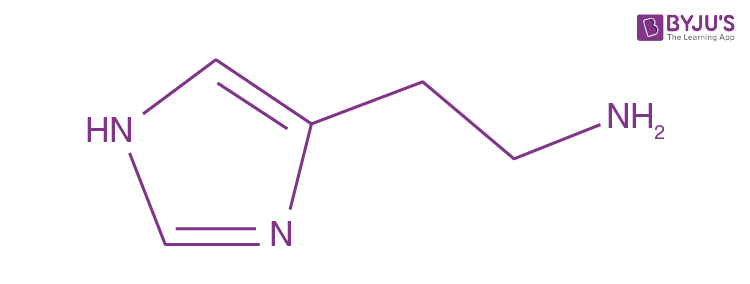### JEE Main 2020 Chemistry Paper January 9 Shift 1